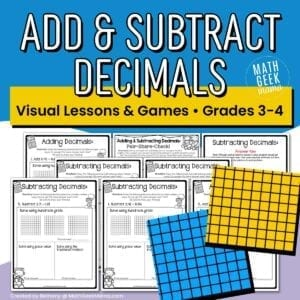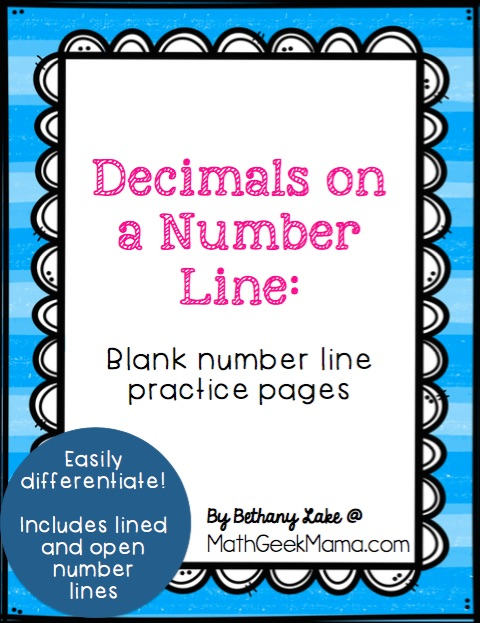# Add & Subtract Decimals on a Number Line {FREE Printable Number Lines!}

Just like fractions, operations with decimals can be intimidating for kids when all they’ve ever known are whole numbers. But with some intentional teaching and strategies, we can help kids understand that the basic underlying principles are the same. Although this should probably not be a starting point (when addition & subtraction with decimals is new), number lines can be a fantastic aid and visual. Read on for how to add & subtract decimals on a number line, and be sure to grab the FREE blank number line practice pages at the end!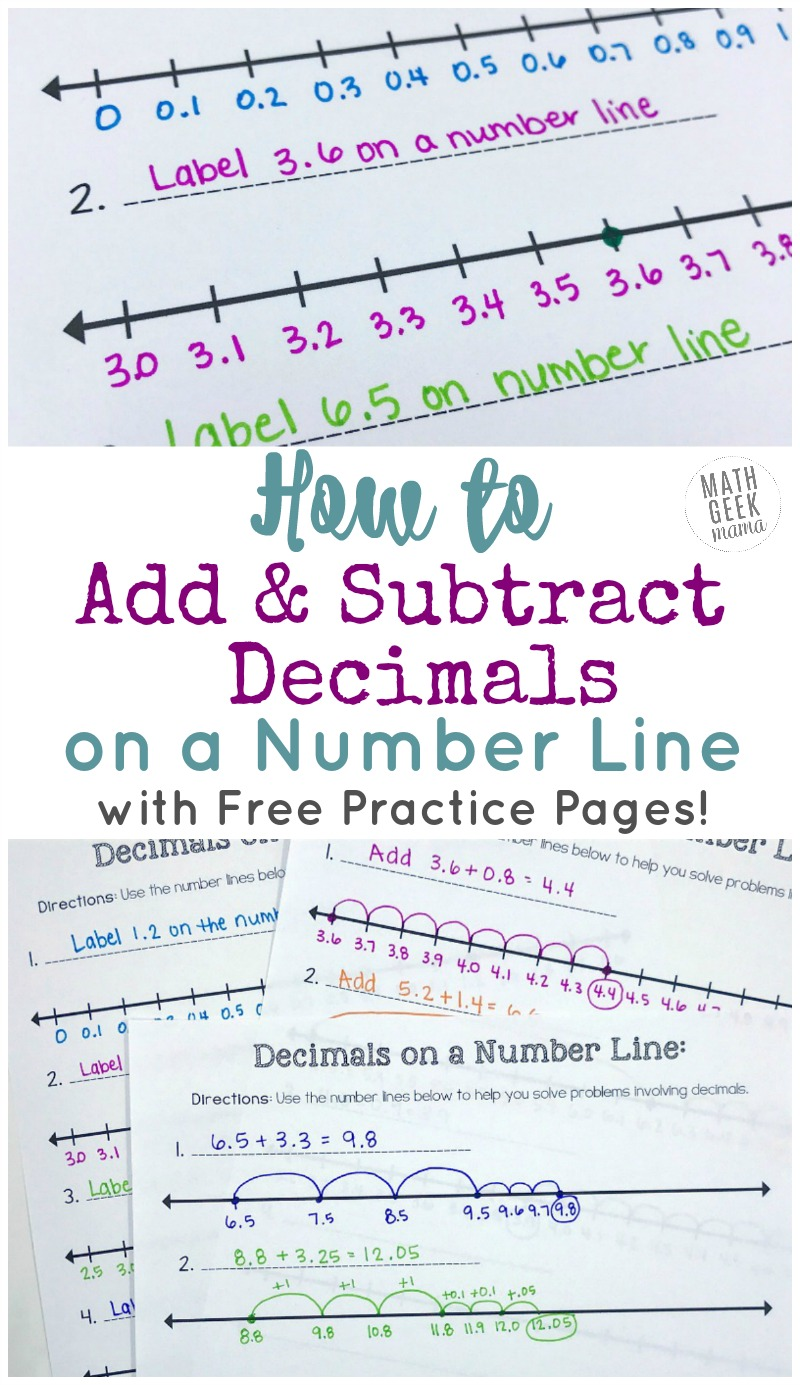*Please Note: This post contains affiliate links which help support the work of this site. Read our full disclosure here.*

## Add & Subtract Decimals with Real World Examples

When you’re first beginning a study of decimal operations, I recommend starting with money. This is a natural and familiar use of decimals, and one kids will be familiar with already.

They may not know how to formally write out the problems, or how to use and write out formal algorithms, but they likely already know how to add up all their dollars and change.

This lesson, with variations for increasing difficulty, is a great place for kids to start.

## Add & Subtract Decimals with Base Ten Blocks

I would then recommend building decimal quantities and modeling addition and subtraction with base ten blocks.

When using base ten blocks for decimals (rather than whole numbers), the hundred block represents one whole and the tens rods represent tenths and then the ones blocks represent hundredths.

This will not only give kids a visual representation of decimal numbers, but reinforce their understanding of place value.

I would begin by giving kids different decimal numbers and having them build it with blocks.

Then you can begin adding whole numbers to show that the decimal portion doesn’t change, and vice versa.

And just as with whole numbers, regrouping works the same way and is easily modeled with base ten blocks.

For more information and examples, see this post from Rachel at You’ve Got This Math.

## Add & Subtract Decimals on a Number Line

Once kids have a solid foundation and understand what decimals numbers represent, you can show them how to use a number line to solve addition & subtraction problems.

Before beginning, start by practicing labeling a number line with decimals rather than whole numbers.

I recommend starting by labeling increments of 0.1.

Label some number lines by starting at 0, and then practice with different starting values, but always labeling increments of 0.1.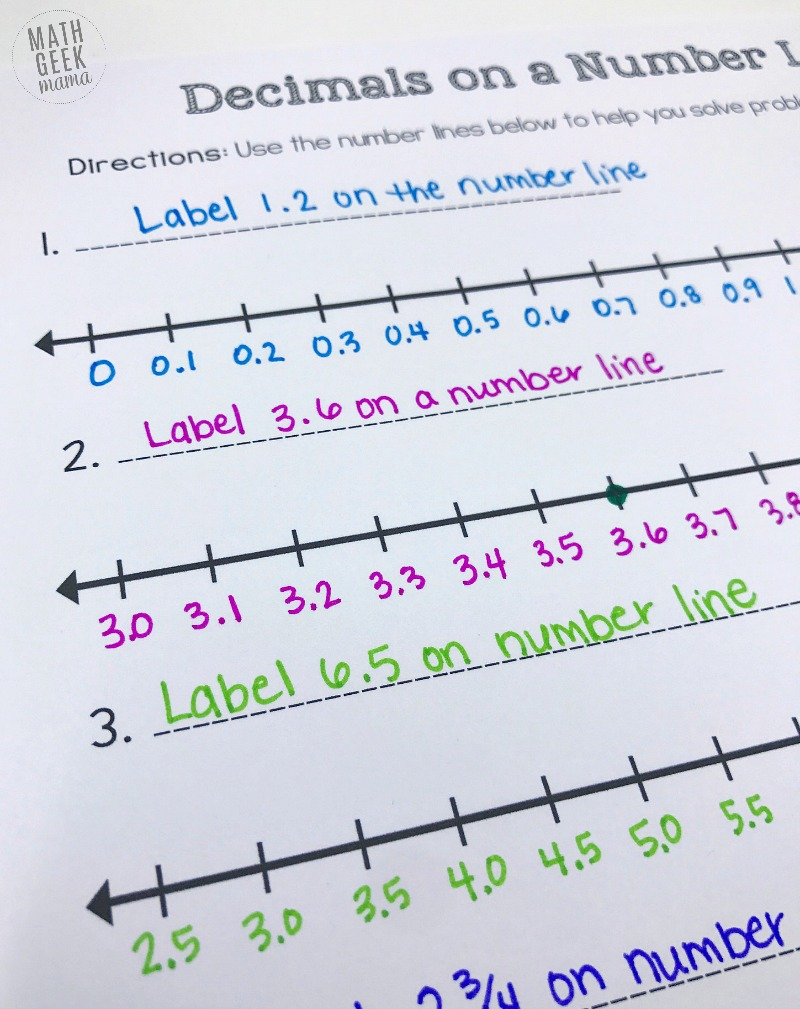Then you can challenge kids to label increments of 0.25 or 0.5. Once they have a few number lines labeled correctly, see if they can find and mark certain values on their number lines.

You can also give them fractions, and have them mark the decimal form on their number line.

Being familiar with how number lines work and how to find points and move around will be essential to actually using a number line to solve a math problem.

But once your kids are comfortable with them, you can give them decimal addition problems to solve.

### Adding Decimals on a Number Line:

For example, let’s say you give kids the problem 3.6 + 0.8.

They would begin by labeling their number line with the starting value (3.6) and then labeling all the way across by tenths.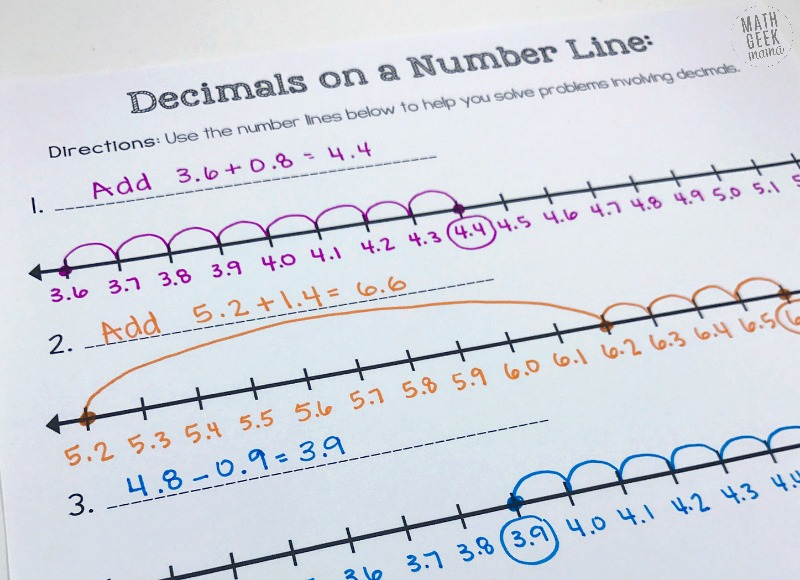Once the number line is labeled, they can simply add on by counting 8 tenths. They mark the number line by showing their “jumps” as they count.

Once they’ve counted 8, they have found their answer.

When adding decimals that include whole numbers, they can begin by adding the whole number first.

For example, in the problem 5.2 + 1.4, kids can jump 1 whole (or ten tenths) to the number 6.2.

Then they can add 0.4 by jumping or counting by tenths and end on the answer: 6.6.

### Subtracting Decimals on a Number Line

With subtraction problems, kids can solve essentially the same way. The difference here is that they will want to start on the far right side of their number line and label it backwards by tenths so that they can subtract.

For example, given the problem 4.8 – 0.9, kids would start by labeling 4.8.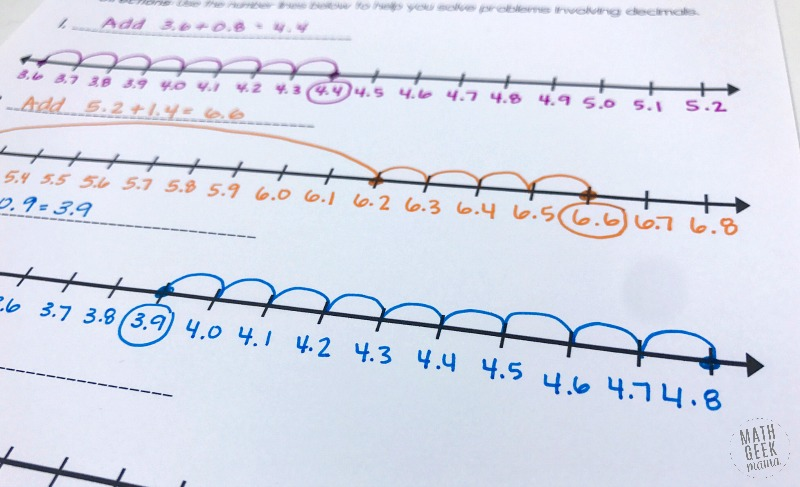They then label backwards to fill in the number line.

To subtract, they simply count backwards by tenths 9 times until the reach the solution: 3.9.

Then as kids encounter more challenging problems, they can label their number lines accordingly and then either add or subtract.

## Add & Subtract Decimals with an Open Number Line

Once kids are more confident working with both number lines and decimals, using an open number line may be more beneficial for them.

An open number does not have any line markers to delineate number values. It is simply a straight line.

With an open number line, kids can start wherever they’d like without having to worry about equal increments.

They can then use it to merely show their thinking as they add or subtract each problem bit by bit. This is especially useful if they have a good understanding of place value.

For example, to add 6.5 + 3.3, kids can start at 6.5 and add 3, using larger “jumps” to show whole numbers. They “land” at 9.5.Then they can count on with smaller jumps to add the tenths. Now they’re at 9.8, the final solution.

Open number lines are especially helpful if kids are adding larger quantities, as there may not be adequate space on a number line that already has tenths marked.

It’s also helpful as they begin to add and subtract hundredths, as they don’t have to worry about finding the correct location on the number line, they can simply add each place value one at a time.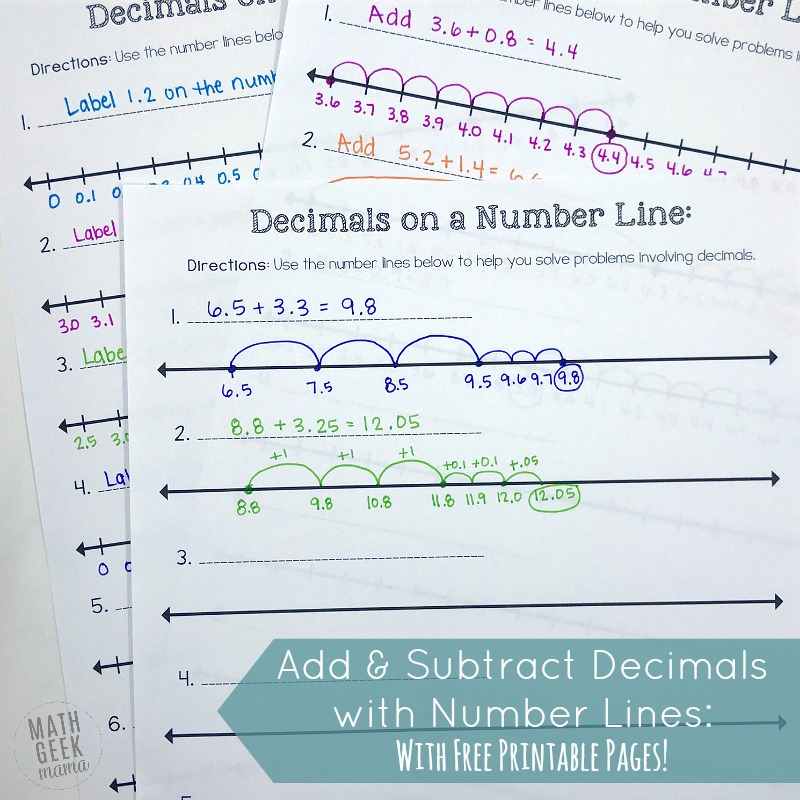Using an open number line to aid and model their thinking is also a great way to help kids work towards mental math with decimals.

Want an easy practice page your kids can use to solve any decimal problem? This simple set includes 2 pages of number lines.

The first is marked with lines so you can practice labeling different increments and help kids to be familiar.

The second is a page of open number lines.

There is also a quick overview page for the teacher with a few different ways you might use these number line pages and teach these concepts to your students.

Looking for more practice with addition & subtraction of decimals? You might like the complete collection of visual lessons and games to help kids make sense!